# INDEX Function – Examples in Excel, VBA, & Google Sheets

This Tutorial demonstrates how to use the Excel INDEX Function in Excel to return a value based on column and row references.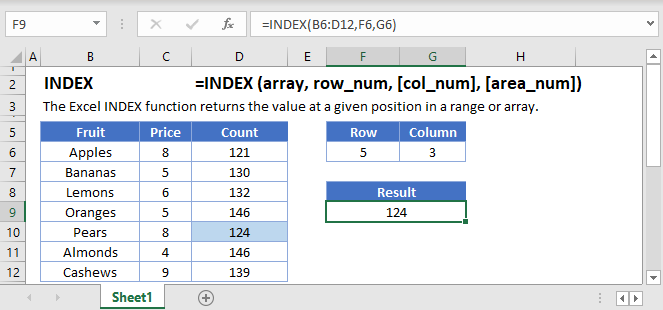## INDEX Function Overview

The INDEX Function Returns a cell value from a list or table based on it’s column and row numbers.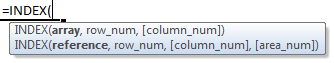## INDEX Function syntax and Argument

array -A range of cells, or an array constant.

row_num – The row position in the reference or array.

column_num – [optional] The column position in the reference or array.

area_num – [optional] The range in reference that should be used.

### What is the INDEX function?

The INDEX function is one of the more versatile functions in the world of spreadsheets, and it can be used in a multitude of ways and increasing level of complexities. As such, we will be discussing the INDEX function over several articles. This article will focus on an introduction to the INDEX function. In simple terms, the INDEX function allows you to return a reference to a specified cell or range of cells.

### Basic example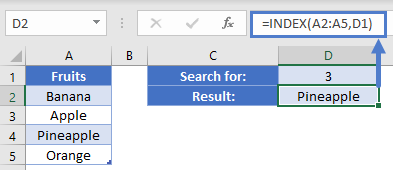We want to be able to tell our formula to choose a specific item from the list based on position. Let’s assume we want the 3rd item from the list. In D1, we put the number 3. In D2, we put the formula

Since D2 is 3, our INDEX is going to return the 3rd cell in this range, which is A4. The value of A4 is “Pineapple” and thus we see that result.

### Table example

Let’s build a multiplication table that looks like this: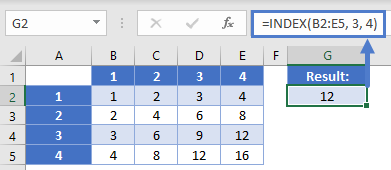To get the result of 3 times 4 (and not just write the math equation), you could write a formula of

In this scenario, we’ve told the formula to go to the 3rd row within the specified range, and the 4th column. This is going to give a reference to cell E4, and we thus get a value of 12.

While using INDEX here to do multiplication is a bit redundant, you could also use this to return text strings.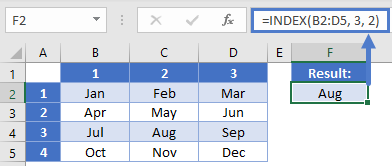In this case, we could say we’re looking for the 2nd month in the 3rd quarter. Our formula would be

Here, our formula goes down to the 3rd row (for the 3rd quarter) and the 2nd column. That returns a reference to C4 and the result of “Aug”.

### Using with MATCH

The INDEX function is often used in conjunction with the MATCH function, who returns the relative position of an item. Let’s look at a grade book example where our teacher wants to be able to give a subject and student name and find the corresponding grade.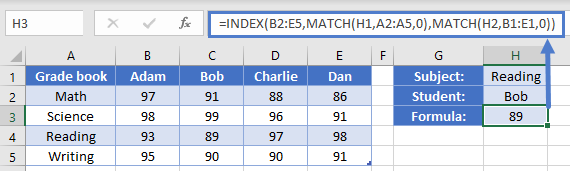Our overall formula is going to be:

We’ve used two different MATCH function to return the relative position of each of our search terms. The first one is looking for the value of H1, “Reading”, in the range of A2:A5. Reading is the 3rd item in the list. Our 2nd MATCH is similarly looking for the value of “Bob” within the range B1:E1. Bob is the 2nd item in this list. Thus, our formula will be evaluated like so:

We will discuss this more in <link to other article(s)>.

The INDEX Function works exactly the same in Google Sheets as in Excel: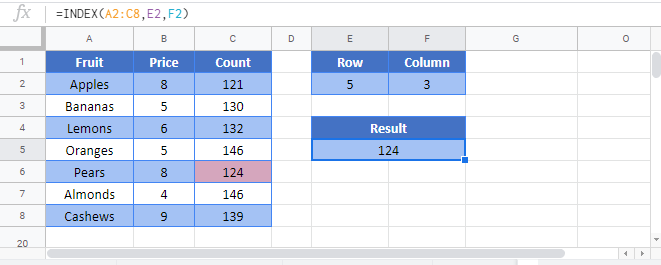Use the INDEX Function to return a value based on it’s position in a range of cells.

First define the range of cells from which to select. Next define the row and column numbers within the array.

You can use the MATCH Function with the INDEX Function to simulate a VLOOKUP Formula with the added benefit that the lookup column(row) does not need to be the leftmost(top) column(row).

## INDEX Examples in VBA

You can also use the INDEX function in VBA. Type:
`application.worksheetfunction.index(array,row_num,column_num)`
For the function arguments (array, etc.), you can either enter them directly into the function, or define variables to use instead.

Assuming we have the following data in our worksheet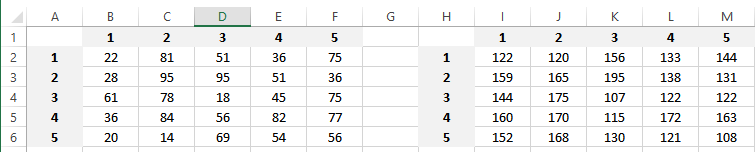There are two tables defined in this example, one table on the left which is named Table2 and on the right Table3

We can use the INDEX function as following

This will return 18 (Cell D4), because it is the cell located at the third row and third column cell in our defined range B2:F6

This will return 51 (Cell E3), because it is the cell located at the second row and fourth column in Table2

We can also define more that one ranges, as following.Notice that we are using one more parameter this time (the last “1”), which defines the range from which the value should be returned

This will return 36 (Cell F3), because it is the cell located at the second row and fifth column in our first defined range, which is Table2

This will return 115 (Cell K5), because it is the cell located at the fourth row and third column in our second defined range, which is I2:M6